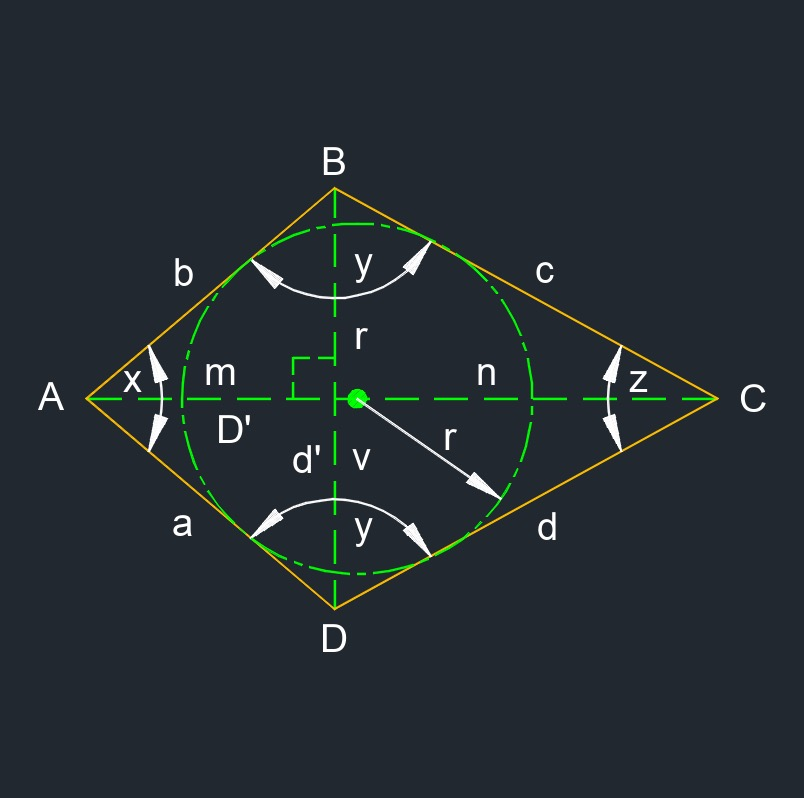# Kite

Written by Jerry Ratzlaff on . Posted in Plane Geometry

•Kite (a two-dimensional figure) is a quadrilateral with two pairs of adjacent sides that are congruent.
• Acute angle measures less than 90°.
• Congruent is all sides having the same lengths and angles measure the same.
• Diagonal is a line from one vertices to another that is non adjacent.
• Obtuse angle measures more than 90°.
• a = b
• c = d
• ∠B = ∠D
• ∠A ≠ ∠C
• ∠A + ∠B + ∠C + ∠D = 360°
• 2 diagonals
• 4 sides
• 4 vertexs

## Angle of a Kite formulas

 $$\large{ x = arccos \; \frac{ m^2 \;+\; a^2 \;-\; \left( \frac{d'}{2} \right)^2 }{ 2\;m\;a } }$$ $$\large{ y = \frac{360° \;-\; x \;-\; z}{2} }$$ $$\large{ z = arccos \; \frac{ \left( D'\;m \; \right)^2 \;+\; d^2 \;-\; \left( \frac{d'}{2} \right)^2 }{ 2\;\left( D'\;m \right)\;d } }$$

### Where:

$$\large{ x }$$ = acute angle

$$\large{ y }$$ = obtuse angle

$$\large{ z }$$ = acute angle

$$\large{ d' }$$ = diagonal

$$\large{ D' }$$ = diagonal

$$\large{ m }$$ = diagonal section

$$\large{ a, b, c, d }$$ = edge

## Area of a Kite formulas

 $$\large{ A_{area} =\frac { d' \;D' }{2} }$$ $$\large{ A_{area} =\frac{1}{2} \; n \; r }$$

### Where:

$$\large{ A_{area} }$$ = area

$$\large{ d' }$$ = diagonal

$$\large{ D' }$$ = diagonal

$$\large{ m, n, r, v }$$ = diagonal

## Diagonal of a Kite formulas

 $$\large{ d' = 2\; \frac {A} {D'} }$$ $$\large{ D' = 2\; \frac {A} {d'} }$$

### Where:

$$\large{ d' }$$ = diagonal

$$\large{ D' }$$ = diagonal

$$\large{ A_{area} }$$ = area

## Edge of a Kite formulas

 $$\large{ a = \frac {p} {2} - c }$$ $$\large{ c = \frac {p} {2} - a }$$

### Where:

$$\large{ a, b, c, d }$$ = edge

$$\large{ p }$$ = perimeter

## Perimeter of a Kite formulas

 $$\large{ p= 2\; \left( a + c \right) }$$ $$\large{ p= 2\;a + 2\;c }$$

### Where:

$$\large{ p }$$ = perimeter

$$\large{ a, b, c, d }$$ = edge